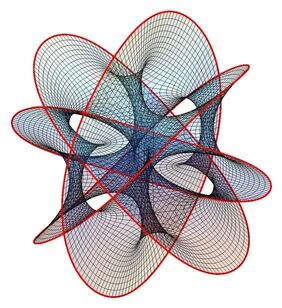Select Page

# Course on K3 Surfaces

Date

Fridays from 10:30 to 13:00 (October 4th to November 29th, 2019)

Location

Lecturer: Martí Lahoz and Joan Carles Naranjo,  University of Barcelona

Course Description:

The main goal of this 20 hours course is to give an introduction to the theory of K3 surfaces, which is a rich and fundamental topic in algebraic geometry touching many other areas as arithmetic, complex and differential geometry, homological algebra, and even mathematical physics. Surfaces with trivial canonical bundle occupy a special place in the classification of 2-dimensional varieties. There are two types of such surfaces: Abelian surfaces and K3 surfaces. On the one hand, Abelian surfaces may be viewed as a straightforward two dimensional analogue of elliptic curves. In particular, Abelian surfaces have a commutative nontrivial fundamental group, while K3 surfaces arise as a new class of simply connected algebraic varieties. Namely, from the complex geometry point of view, they are the lowest dimensional examples of either hyperkähler manifolds or strict Calabi-Yau manifolds.

The course is organized as follows: in the first block we will provide some basics which are universal tools in many geometric theories: Kähler manifolds, sheaves and Hodge theory. In the second part we will focus in the classical theory of K3 surfaces which culminates in the famous Torelli theorem. Finally, depending of the interest of the audience, we will move to some more recent aspects, showing that the study of K3 surfaces has motivated the development of many powerful tools in each field.

Organiser:  Joan Carles Naranjo (Universitat de Barcelona)Date

Fridays from 10:30 to 13:00 (October 4th to November 29th, 2019)

Location

Faculty of Mathematics and Computer Science – University of Barcelona

Lecturer: Martí Lahoz and Joan Carles Naranjo,  University of Barcelona

Course Description:

The main goal of this 20 hours course is to give an introduction to the theory of K3 surfaces, which is a rich and fundamental topic in algebraic geometry touching many other areas as arithmetic, complex and differential geometry, homological algebra, and even mathematical physics. Surfaces with trivial canonical bundle occupy a special place in the classification of 2-dimensional varieties. There are two types of such surfaces: Abelian surfaces and K3 surfaces. On the one hand, Abelian surfaces may be viewed as a straightforward two dimensional analogue of elliptic curves. In particular, Abelian surfaces have a commutative nontrivial fundamental group, while K3 surfaces arise as a new class of simply connected algebraic varieties. Namely, from the complex geometry point of view, they are the lowest dimensional examples of either hyperkähler manifolds or strict Calabi-Yau manifolds.

The course is organized as follows: in the first block we will provide some basics which are universal tools in many geometric theories: Kähler manifolds, sheaves and Hodge theory. In the second part we will focus in the classical theory of K3 surfaces which culminates in the famous Torelli theorem. Finally, depending of the interest of the audience, we will move to some more recent aspects, showing that the study of K3 surfaces has motivated the development of many powerful tools in each field.

Organiser:  Joan Carles Naranjo (Universitat de Barcelona)

##### Contents

(1) Preliminaries
(a) Kähler manifolds.
(b) Sheaves and cohomology.
(c) Hodge decomposition.
(d) Intersection theory on surfaces.
(2) K3 surfaces.
(a) Definition and examples.
(b) Hodge structure on K3 surfaces.
(c) Projectivity of surfaces and GAGA theorems.
(d) Periods.
(e) Torelli Theorem.
(3) Different directions from here
(a) Moduli spaces of sheaves on K3 surfaces. Hyperkähler goeometry.
(b) Automorphisms on K3 surfaces. Dynamics in K3 surfaces.
(c) K3 surfaces and arithmetic. Elliptic K3 surfaces. Rational points and rational curves.
(d) Derived categories.

##### References

[Bea96] Arnaud Beauville. Complex algebraic surfaces, volume 34 of London Mathematical Society Student Texts. Cambridge
University Press, Cambridge, second edition, 1996. Translated from the 1978 French original by R. Barlow,
with assistance from N. I. Shepherd-Barron and M. Reid.

[BHPVdV04] Wolf P. Barth, Klaus Hulek, Chris A. M. Peters, and Antonius Van de Ven. Compact complex surfaces, volume 4 of
Ergebnisse der Mathematik und ihrer Grenzgebiete. 3. Folge. A Series of Modern Surveys in Mathematics [Results in
Mathematics and Related Areas. 3rd Series. A Series of Modern Surveys in Mathematics]. Springer-Verlag, Berlin,
second edition, 2004.

[Can01a] S. Cantat. Sur la dynamique du groupe d’automorphismes des surfacesK3. Transform. Groups, 6(3):201–214, 2001.

[Can01b] Serge Cantat. Dynamique des automorphismes des surfaces K3. Acta Math., 187(1):1–57, 2001.

[HL10] Daniel Huybrechts and Manfred Lehn. The geometry of moduli spaces of sheaves. Cambridge Mathematical Library.
Cambridge University Press, Cambridge, second edition, 2010.

[Huy05] Daniel Huybrechts. Complex geometry. Universitext. Springer-Verlag, Berlin, 2005. An introduction.

[Huy06] D. Huybrechts. Fourier-Mukai transforms in algebraic geometry. Oxford Mathematical Monographs. The Clarendon
Press, Oxford University Press, Oxford, 2006.

[Huy16] Daniel Huybrechts. Lectures on K3 surfaces, volume 158 of Cambridge Studies in Advanced Mathematics. Cambridge
University Press, Cambridge, 2016.

[McM02] Curtis T. McMullen. Dynamics on K3 surfaces: Salem numbers and Siegel disks. J. Reine Angew. Math., 545:201–
233, 2002.

[Sch13] Matthias Sch¨utt. Two lectures on the arithmetic of K3 surfaces. In Arithmetic and geometry of K3 surfaces and
Calabi-Yau threefolds, volume 67 of Fields Inst. Commun., pages 71–99. Springer, New York, 2013.

[Voi07] Claire Voisin. Hodge theory and complex algebraic geometry. I, volume 76 of Cambridge Studies in Advanced
Mathematics. Cambridge University Press, Cambridge, english edition, 2007. Translated from the French by Leila
Schneps.